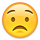# The Net Worth report isn't correctThe Net Wor[th] calculations are wrong. When using a multi-currency portfolio, the net worth converts all the currencies to 1 vs. the USD. So, if one has an asset in yen, its value in the net worth calculations is multiplied by a 100 approximately. So end value of the net worth is totally wrong.
The net worth by periods (year, quarter, etc.) doesn't aggregate the values but rather gives the summary by currency independently from each other. So, the total is correct but no aggregate number.
Does someone knows if Quicken is working on addressing these issues for the upcoming updates?
Tagged: